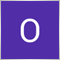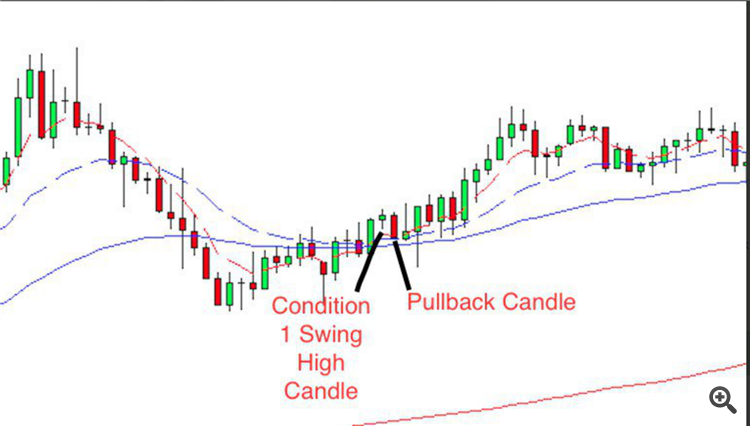# How to create multiple condition in 1 Custom Indicator59

Hey guys,

Just wondering anyone able to offer me some help. Basically I have done the coding of below condition.

1st Condition, I saw a crossover between two different moving averages and the candle that appeared I named it as Swing High Candle.

2nd Condition, I would like to see a insideBar/pullback candle after the Swing High Candle. (refer to the picture)

My question is, I have done the 1st condition coding, I got no idea where should I slot in my 2nd condition. Or how it shall be called, at least when the alert sound, I shall know it meet two condition instead of one of it.```#property copyright "Copyright 2018"
#property version   "1.00"

#property indicator_chart_window
#property indicator_buffers 3
#property indicator_color1 clrGreen //CrossoverUp
#property indicator_color2 clrRed //CrossoverDown
#property indicator_color3 clrBlue //InsideBar
#property indicator_width1 2 //CrossoverUp
#property indicator_width2 2 //CrossoverDown
#property indicator_width3 2 //InsideBar

extern string              Crossover_Alert="This is Crossover Column!";
extern int                 MAPeriod1 =    5;
extern ENUM_MA_METHOD      MAMethod1=MODE_EMA;
extern int                 MAPeriod2 =    6;
extern ENUM_MA_METHOD      MAMethod2=MODE_EMA;
extern int                 MAPeriod3 =    0;
extern ENUM_MA_METHOD      MAMethod3=MODE_EMA;
extern int                 MAPeriod4 =    0;
extern ENUM_MA_METHOD      MAMethod4=MODE_SMA;
double                     CrossUp[];
double                     CrossDown[];

//--------------------------InsideBar Start--------------------------
extern bool          Display_Inside_Bar=true;

datetime                   candletime=0;
datetime                   currenttime=0;
double               val1[];

int init()
{
//---- Indicator Start ----
SetIndexStyle  (0, DRAW_ARROW, EMPTY); //CrossoverUp
SetIndexArrow  (0, 233);
SetIndexBuffer (0, CrossUp);

SetIndexStyle  (1, DRAW_ARROW, EMPTY); //CrossoverDown
SetIndexArrow  (1, 234);
SetIndexBuffer (1, CrossDown);

SetIndexStyle     (2,DRAW_HISTOGRAM,0,1);
SetIndexBuffer    (2,val1);
SetIndexStyle     (2,DRAW_ARROW);
SetIndexArrow     (2,84);
//---- Indicator End ----

return(0);
}

int deinit()
{
ObjectDelete(OBJ_NAME);
return(0);
}

int start()
{
//--------------------------Crossover Declaration Start--------------------------
int                  limit;
int                  counted_bars=IndicatorCounted();
double               Range, AvgRange;
//--------------------------Crossover Declaration End--------------------------

//--------------------------InsideBar Declaration Start--------------------------
double            Range1, AvgRange1, O, O1, C, C1, L, L1, H, H1;
static datetime   prevtime = 0;
string            pattern, period;
//--------------------------InsideBar Declaration End--------------------------

if(prevtime == Time)
{
return(0);
}
prevtime = Time;

switch (Period()) {
case 1:
period = "M1";
break;
case 5:
period = "M5";
break;
case 15:
period = "M15";
break;
case 30:
period = "M30";
break;
case 60:
period = "H1";
break;
case 240:
period = "H4";
break;
case 1440:
period = "D1";
break;
case 10080:
period = "W1";
break;
case 43200:
period = "MN";
break;
}
for (int j = 0; j < Bars; j++) {

}

for (shift = 0; shift < Bars; shift++) {
counter1=shift;
Range1=0;
AvgRange1=0;
for (counter1=shift ;counter1<=shift+9;counter1++) {
AvgRange=AvgRange+MathAbs(High[counter1]-Low[counter1]);
}

Range1=AvgRange1/9;
shift1 = shift ;
shift2 = shift + 1;

O = Open[shift1];
O1 = Open[shift2];
H = High[shift1];
H1 = High[shift2];
L = Low[shift1];
L1 = Low[shift2];
C = Close[shift1];
C1 = Close[shift2];

// Check for In pattern
if ((H<H1)&&(L>L1))
{
if (Display_Inside_Bar == true)
{
string val2 = True;
val1[shift]=High[shift];
}
}
}

//--------------------------Crossover & Inside Bar Coding Start--------------------------
currenttime=Time;
if(counted_bars<0) return(-1); //---- check for possible errors
if(counted_bars>0) counted_bars--;//---- last counted bar will be recounted

limit=Bars-counted_bars;

for(int i=0;i<=limit;i++)
{
int counter=i;
Range=0;
AvgRange=0;
for (counter=i ;counter<=i+9;counter++)
{
AvgRange=AvgRange+MathAbs(High[counter]-Low[counter]);
}
Range=AvgRange/10;

for(i=0;i<=limit;i++)
{
//-------------Declaration on current Time Frame Start----------------------------
double ma1           =iMA(NULL,0,MAPeriod1,0,MAMethod1,PRICE_CLOSE,i); //6
double ma1P          =iMA(NULL,0,MAPeriod1,0,MAMethod1,PRICE_CLOSE,i+1);
double ma1A          =iMA(NULL,0,MAPeriod1,0,MAMethod1,PRICE_CLOSE,i-1);

double ma2           =iMA(NULL,0,MAPeriod2,0,MAMethod2,PRICE_CLOSE,i); //18
double ma2P          =iMA(NULL,0,MAPeriod2,0,MAMethod2,PRICE_CLOSE,i+1);
double ma2A          =iMA(NULL,0,MAPeriod2,0,MAMethod2,PRICE_CLOSE,i-1);

double ma3           =iMA(NULL,0,MAPeriod3,0,MAMethod3,PRICE_CLOSE,i); //50
double ma4           =iMA(NULL,0,MAPeriod4,0,MAMethod4,PRICE_CLOSE,i); //200
//-------------Declaration on current Time Frame End----------------------------
if(ma1>ma2 && ma1P<ma2P && ma1A>ma2A && ma1>ma3 && ma2>ma3 && ma3>ma4)
{
CrossUp[i] = Low[i] - Range*0.6;
if(currenttime!=candletime && i==1)
{
}
candletime=Time; //Current Time is Time;
}
}
else if(ma1<ma2 && ma1P>ma2P && ma1A<ma2A && ma1<ma3 && ma2<ma3 && ma3<ma4)
{
CrossDown[i] = High[i] + Range*0.6;
if(currenttime!=candletime && i==1)
{